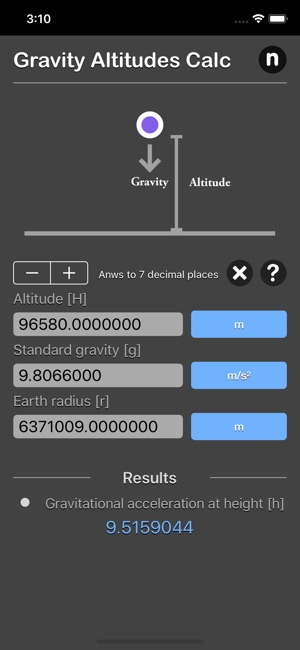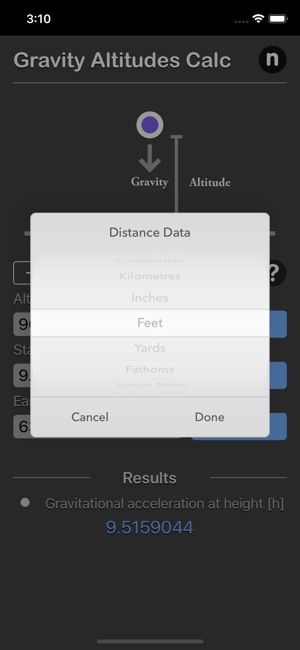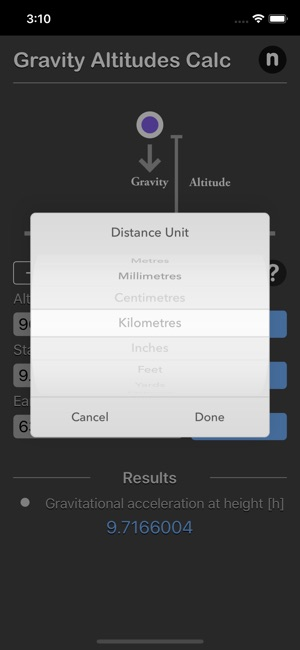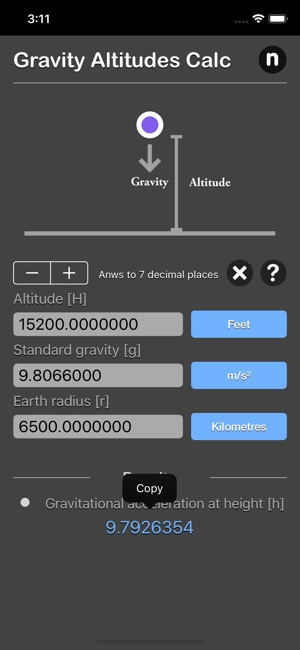## Screenshots

••••••## Description

Gravity Altitudes Calculator are physic/math calculator to find the acceleration due to gravity at altitude or height [h] from the surface of the Earth fast and easy.

Features:
- Instant calculation
- Result are copy able to other app
- Formula are include as reference
- Support up to 16 decimal place
- Support various unit for each input

The gravity of Earth, which is denoted by g, refers to the acceleration that the Earth imparts to objects on or near its surface due to gravity.
In SI units this acceleration is measured in metres per second squared (in symbols, m/s² or m·s⁻²).
Gravity of Earth has an approximate value of 9.81 m/s², which means that, ignoring the effects of air resistance, the speed of an object falling freely near the Earth’s surface will increase by about 9.81 metres (32.2 ft) per second every second.

The Acceleration due to Gravity by Altitude formula computes the approximate acceleration due to gravity on the surface of the Earth based on the altitude.
Formula:
gh = g0 x (re / re + h)²

Where:
gh - gravitational acceleration at height [h] above sea level. (m/s² )
g0 - Standard gravity
re - Earth's mean radius. (km)
h - altitude, height above the surface at which the value of acceleration is to be measured.

The Formula uses two constants:
Gravity Acceleration on the surface of Earth = 9.80665 m/s²
Mean Radius of Earth=6371.009 km

*This is a universal app that work for both iPhone and iPad.

Thanks for your support and do visit nitrio.com for more apps for your iOS devices.

## Information

Seller
Tan Chia Ling
Size
16.4 MB
Category
Utilities
Compatibility

Requires iOS 8.0 or later. Compatible with iPhone, iPad, and iPod touch.

Languages

English

Age Rating
Rated 4+
•